# infinite graph

## Characterizing (aleph_0,aleph_1)-graphs ★★★

Call a graph an-graph if it has a bipartition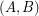so that every vertex inhas degreeand every vertex inhas degree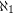.

Problem   Characterize the-graphs.

## Highly arc transitive two ended digraphs ★★

Author(s): Cameron; Praeger; Wormald

Conjecture   Ifis a highly arc transitive digraph with two ends, then every tile ofis a disjoint union of complete bipartite graphs.

Keywords: arc transitive; digraph; infinite graph

## Strong matchings and covers ★★★

Author(s): Aharoni

Letbe a hypergraph. A strongly maximal matching is a matching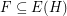so that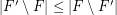for every matching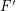. A strongly minimal cover is a (vertex) coverso that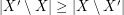for every cover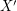.

Conjecture   Ifis a (possibly infinite) hypergraph in which all edges have size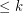for some integer, thenhas a strongly maximal matching and a strongly minimal cover.

Keywords: cover; infinite graph; matching

## Unfriendly partitions ★★★

Author(s): Cowan; Emerson

Ifis a graph, we say that a partition ofis unfriendly if every vertex has at least as many neighbors in the other classes as in its own.

Problem   Does every countably infinite graph have an unfriendly partition into two sets?

Keywords: coloring; infinite graph; partition

## Hamiltonian cycles in powers of infinite graphs ★★

Author(s): Georgakopoulos

Conjecture
\item Ifis a countable connected graph then its third power is hamiltonian. \item Ifis a 2-connected countable graph then its square is hamiltonian.

Keywords: hamiltonian; infinite graph

## Hamiltonian cycles in line graphs of infinite graphs ★★

Author(s): Georgakopoulos

Conjecture
\item Ifis a 4-edge-connected locally finite graph, then its line graph is hamiltonian. \item If the line graphof a locally finite graphis 4-connected, thenis hamiltonian.

Keywords: hamiltonian; infinite graph; line graphs

## Infinite uniquely hamiltonian graphs ★★

Author(s): Mohar

Problem   Are there any uniquely hamiltonian locally finite 1-ended graphs which are regular of degree?

## Unions of triangle free graphs ★★★

Author(s): Erdos; Hajnal

Problem   Does there exist a graph with no subgraph isomorphic to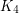which cannot be expressed as a union oftriangle free graphs?

## Seymour's self-minor conjecture ★★★

Author(s): Seymour

Conjecture   Every infinite graph is a proper minor of itself.

Keywords: infinite graph; minor